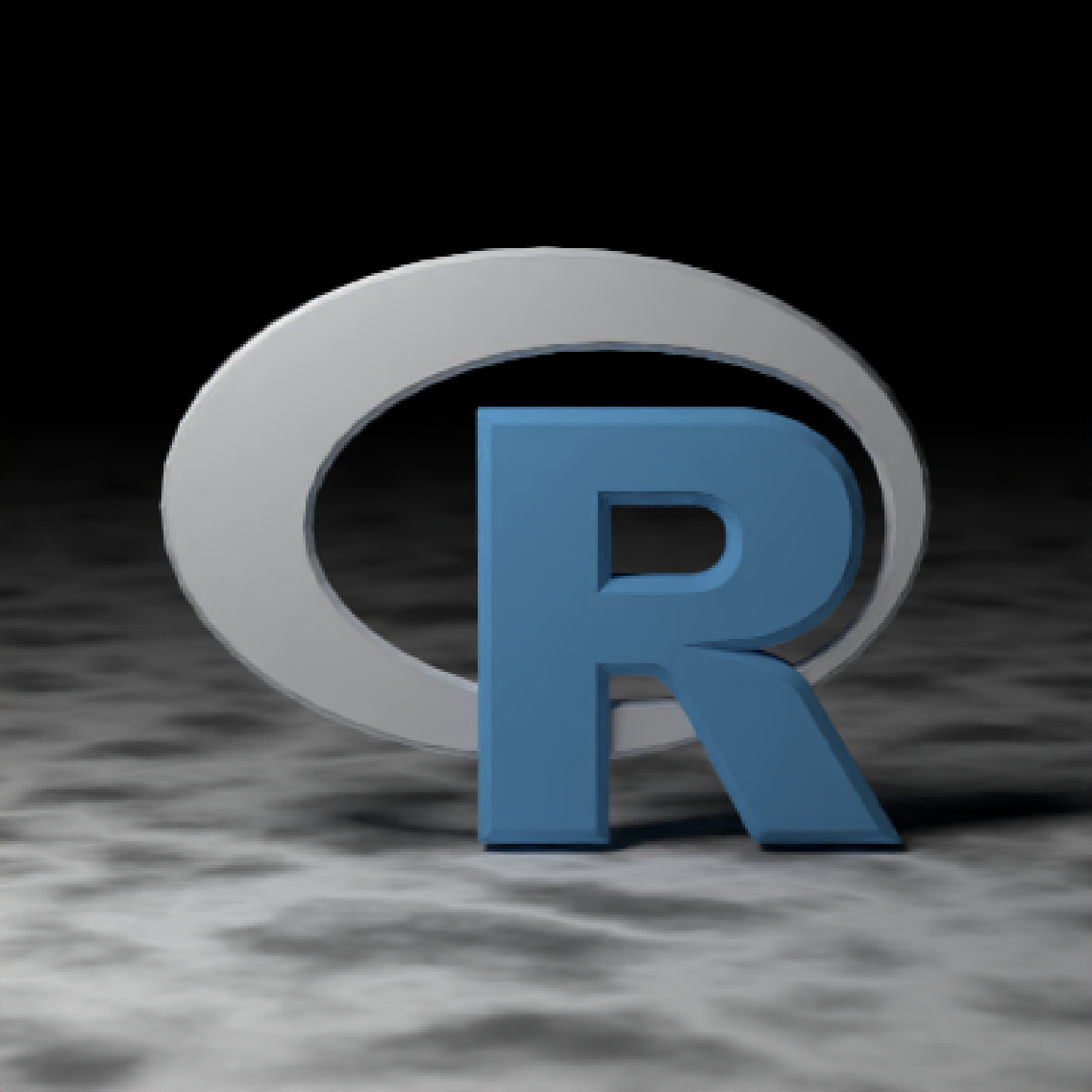3D obj model of the letter R, to be used with obj_model()

r_obj()

## Value

File location of the R.obj file (saved with a .txt extension)

## Examples

#Load and render the included example R object file.
if(rayrender:::run_documentation()) {
generate_ground(material = diffuse(noise = TRUE, noisecolor = "grey20")) %>%
add_object(sphere(x = 2, y = 3, z = 2, radius = 1,
material = light(intensity = 10))) %>%
add_object(obj_model(r_obj(), y = -1, material = diffuse(color="red"))) %>%
render_scene(parallel=TRUE, lookfrom = c(0, 1, 10), clamp_value = 5, samples = 200)
}